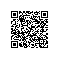# Python机器学习小技巧：GroupBy 和 Shift实现 Pandas分组错位

pandas.DataFrame.shift

DataFrame.shift(self,periods=1,freq=None,axis=0,fill_value=None)[source]

Shift index by desired number of periods with an optional time freq.

When freq is not passed, shift the index without realigning the data. If freq is passed (in this case, the index must be date or datetime, or it will raise a NotImplementedError), the index will be increased using the periods and the freq.

df1['x_pre']=df1.groupby('CARID')['x'].shift(1)

df1['x_next']=df1.groupby('CARID')['x'].shift(-1)

df1['y_pre']=df1.groupby('CARID')['y'].shift(1)

df1['y_next']=df1.groupby('CARID')['y'].shift(-1)使用钉钉扫一扫加入圈子
+ 订阅